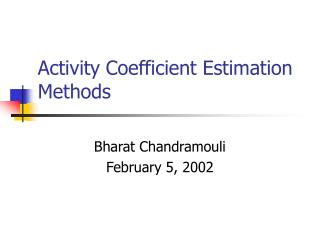DownloadDownload PresentationActivity Coefficient Estimation Methods

# Activity Coefficient Estimation Methods

Download Presentation## Activity Coefficient Estimation Methods

- - - - - - - - - - - - - - - - - - - - - - - - - - - E N D - - - - - - - - - - - - - - - - - - - - - - - - - - -
##### Presentation Transcript

1. Activity Coefficient Estimation Methods Bharat Chandramouli February 5, 2002

2. Activity Coefficient • The activity coefficient is a measure of the non-ideality of mixing • Two components, Enthalpic and Entropic

3. Estimation/Measurement • Activity coefficients in single component/simple mixtures easy to measure • Activity coefficients in water or octanol can be calculated from solubility given sufficiently sensitive methods

4. Need for estimation • What about complex mixtures? • What about dynamic systems with changing compositions? • It becomes more practical to use estimation methods to approximate g in these cases

5. Estimation Methods • Group contribution methods are most common because they have predictive ability • There are two group contribution methods commonly used for iom • calculation from solubility parameters • UNIFAC calculation

6. UNIFAC the UNIversal Functional group Activity Coefficient model • The activity coefficient is calculated from two components Combinational (V, SA) Residual (interactions) (Experiment Fit)

7. UNIFAC • The group contribution components consist of • volume contributor -Rk • surface area contribution -Qk • interaction parameter between functional groups Amk • To calculate interactions, similar sub-groups are assigned to groups and interactions are between these groups • Calculate activity coefficients by summing all contributions and interactions

8. UNIFAC-Simple example • Ethanol CH3-CH2-OH

9. UNIFAC Methods • Interaction parameters are fit from experimental data • This work is still ongoing and many parameters still not available

10. Hansen Solubility Parameter • This method calculates activity coefficients from the solubility parameter • Theory of cohesive energy developed by Hildebrand for dispersive systems and extended by Hansen for polar and hydrogen bonding

11. Hansen Activity Coefficient • The activity coefficient is given by Cohesive energy density Molar Volume Size effect term Enthalpy Entropy

12. The Size Effect Term • i,omd is a measure of the effect of differing sizes of i and om on their entropy of mixing • This was derived by Flory and Huggins using statistical thermodynamics • For an infinitely dilute solution

13. Cohesive Energy (Ecoh) • Closely linked to the heat of evaporation • It is a measure of a the ability of a liquid molecule to stay together • Theory of cohesive energy developed by Hildebrand for dispersive systems and extended by Hansen for polar and hydrogen bonding

14. Solubility parameter • Solubility parameters are measures of cohesive energy cohesive energy solubility parameter coh. energy density

15. Calculating solubility parameters • Hansen and others compiled molar attraction constants for functional groups, which are additive contributions to the solubility parameter

16. Attraction Constants (F) • The product of V was found to vary linearly across homologous series • Additivity of structural sub-groups • F=V values compiled for dispersion and polar components of  • Hansen later compiled additive contributions to Eh

17. Multi-component Mixtures • How are om parameters calculated? • Parameters weighted using component mole fraction and molar volume to get “average om”

18. Cohesive Energy Density • i,omA can be derived as • ib is a weighting factor based on dispersive forces, has been tabulated for a variety of compounds • ib corrects for the fact that polar and H bonding forces are localized

19. Activity Coefficient • Putting the two components together lniom = +

20. Calculation • First, calculate group contributions for each component in the mixture • Calculate “om” parameters by weighting with mole fraction and molar volume • Calculate parameters for compound of interest • Calculate activity coefficient

21. Hansen or UNIFAC? • UNIFAC more powerful interaction • UNIFAC not universal–missing parameters • Hansen has certain inconsistencies as certain parameters have to be culled from different sources. Very sensitive to parameter choice • ib not widely available for many compounds, so estimation may be difficult

22. Where do you use this? • Water solubility estimation • Solvent-Water partitioning (Kow)

23. gas Compound Thermodynamic Equilibrium? Particle type Temperature Humidity particle Gas/Particle Partitioning • What happens when a semivolatile organic (SOC) encounters a particle??

24. Partitioning Modes • Mode of SOC-particle interaction depends on the particle • Adsorption Solid particle, no organic liquid layer (dust, inorganic salts) • Absorption Particle either liquid, or has substantial liquid layer (combustion particles, secondary organic aerosol) • SOCs such as PAHs, and alkanes primarily partition to organic or carbonaceous aerosols rather than to mineral-based aerosols

25. Predictive Partitioning models • Pankow (1994) for absorptive partitioning fom- fraction extractable organic matter igom- activity coefficient of SOC in om MWom-molecular weight of om